Reach Us+44-1904-929220
A Numerical Study of Temperature Profile by Coupling Memory-Based Diffusivity Model With Energy Balance During Thermal Flooding | OMICS International
Journal of Petroleum & Environmental Biotechnology

Like us on:

# A Numerical Study of Temperature Profile by Coupling Memory-Based Diffusivity Model With Energy Balance During Thermal Flooding

Amjed Hassan M and Enamul Hossain M*

Department of Petroleum Engineering, King Fahd University of petroleum & Minerals (KFUPM), Dhahran 31261, Saudi Arabia

*Corresponding Author:
Enamul Hossain M
Department of Petroleum Engineering, King Fahd University of petroleum & Minerals (KFUPM)
Dhahran 31261, Saudi Arabia
Tel: +966 (3) 860 0000
E-mail: [email protected]

Received date: August 04, 2016; Accepted date: September 05, 2016; Published date: September 12, 2016

Citation: Amjed Hassan M, Enamul Hossain M (2016) A Numerical Study of Temperature Profile by Coupling Memory-Based Diffusivity Model With Energy Balance During Thermal Flooding. J Pet Environ Biotechnol 7:300. doi: 10.4172/2157-7463.1000300

Copyright: © 2016 Amjed Hassan M, et al. This is an open-access article distributed under the terms of the Creative Commons Attribution License, which permits unrestricted use, distribution, and reproduction in any medium, provided the original author and source are credited.

Visit for more related articles at Journal of Petroleum & Environmental Biotechnology

#### Abstract

Accurate estimation of the temperature distribution within a reservoir undergoing a thermal recovery operation is a key factor in process design, reservoir management and production forecasting. The thermal and rheological properties of the reservoir rock and fluids play significant roles in the heat transfer between the formation matrix and flowing fluids.

The memory-based diffusivity equation is implemented as a momentum-balance to present continuous alteration of rock and fluid properties and to investigate the temperature propagation during thermal flooding process. This model is coupled with recently developed energy balance equation to investigate the different parameters that influence the temperature profile. Numerical solution of the coupled mathematical model is presented for the case of equal rock and fluid temperature. It is assumed that the rock attains the fluid temperature instantaneously, that is, the rock and fluid temperatures are assumed equal throughout the reservoir. Matlab 7.10 program is used to carry out the computation and provides temperature profiles.

Results show that, coupling the memory-based diffusivity model with energy balance leads to more reasonable temperature profiles during the thermal flooding. The distribution of reservoir temperature with respect to time and distance can be estimated by coupling the memory-based equation and the mathematical tool which were developed by Hossain et al., in addition, it can be concluded that, the fluid velocity, time and the rheological properties, have important effects on the temperature distributions throughout the reservoir. In future, the results of the numerical solution can be integrated with lab experiment results to predict performance of thermal flooding process and better understanding of reservoir management.

#### Keywords

Memory concept; Diffusivity equation; Rock; Fluid interaction; Porous media; Integro-differential equation; Heat transfer coefficient; Peclet number

#### Introduction

The heat transfers from region of higher temperature to lowertemperature region due to the temperature differences in all physical models. This transport process continues until the system attains a uniform temperature. Conduction, radiation, and convection are considered to be the main mechanisms of the heat flux. In any system, conduction, radiation play significant rule in heat transfer, while convective heat transfer is of utmost interest in porous media where fluid velocity is the major concern. In the hydrocarbon reservoirs, the temperature distribution is an important issue due to its utilization in detecting water or gas influx or type of fluid entering into the wellbore, therefore, it will enhance the better understanding of reservoir management and future prediction of the performance of reservoir. During thermal EOR operations, the highly complex characteristics of rock–fluid interaction play a vital role in heat transfer between the rock matrix and flowing hydrocarbons. Such heat transfer is the major factor governing the temperature profile within the reservoir. It is very important to understand the complex rheology of fluid flowing through formation rock. The most challenging issues during the development of models are the time dependency of the rock and fluid properties and the scale of time. Therefore, the notion of memory can be introduced to model the variations of rock and fluid properties with time. In addition, memory term can be used to analyze the rheological behavior of rock and fluid properties when the rock is not in thermal equilibrium with the fluid(s) .

Hossain et al.  demonstrated extensive studies of fluid memory concept on the available literature and models. They presented that different fluid properties such as stress or density have been used to identify the fluid memory concept. Caputo  modified Darcy’s law to consider the memory concept by introducing the order of the fractional derivative (α) and the pseudo-permeability ratio of medium with memory to fluid viscosity (η). These two parameters have been introduced instead of formation permeability and fluid viscosity in conventional Darcy’s law to simulate the effects of changing permeability and viscosity over time. Zhang  defined memory concept as a function of time and space as the forward time events is dependent on previous time events.

In the application of porous media, Iaffaldano et al.  investigated the permeability variations with time in sand formations; they developed a memory model for flowing of fluids in porous formation, their model matches with the experimental results. They concluded that the flux rate variations observed during the experiments were compatible with the compaction of sand. This variation was due to the amount of fluid that went through the grains locally. As a result, there was a reduction of porosity.

Hossain [6,7] developed a new porous media diffusivity equation with the inclusion of rock and fluid memories by invoking timedependent viscosity and permeability. The developed model is highly non-linear and has an option of considering the memory variables. The non-linear solution of this model shows the difference between the predication of the classical diffusivity equation and the proposed memory- based model. The results show that the proposed model could be used for a wide range of applications. Their work establishes the contribution of memory effect on the fluid flow through the reservoir formations.

Zavala-Sanchez et al.  conducted dynamic model to study the effective mixing and spreading dynamics at preasymptotic times in terms of effective average transport coefficients. They found that, the system remembers its initial state, which was defined as memory effects for the effective transport coefficients.

Giuseppe et al.  proposed a modified constitutive equation, the introduced the memory formalism on both the flux term and the pressure gradient variations. They modelled the memory effect by using the fractional order derivatives. In addition, they performed three laboratory experiments to validate their model, homogeneous and heterogeneous formations with different particle size were employed. Their model successfully provides an efficient and useful mean to quantify the memory effects.

Hossain et al.  studied the memory effect and pressure variations on the stress-strain relationship. They obtained the variation of shear stress as a function of strain rate for crude oil to investigate the effects of fluid memory. They found that, the fluid memory causes nonlinearity, which leads to chaotic behaviour of the stress-strain relationship. Their model can be used in rheological study and reservoir simulation; also it can be used to select the proper surfactant or foam for EOR processes.

During the enhanced oil recovery processes, the fluid flows through a porous medium and this will lead to alteration of rock and fluid properties during thermal recovery [11-14]. The formation permeability and porosity may vary locally with time due to several reasons such as chemical dissolution of the medium, swelling and flocculation, pore plugging and fine precipitations [5,9,15]. Most importantly, the alteration of rock and fluid properties controls the temperature distribution within the reservoir formation. Yoshioka et al. [16,17] found that, the fluid velocity and time have strong effects on the temperature profile. Therefore, it is important to investigate the effects of memory on the rheology of the rock–fluid system .

Hossain et al.  studied the role of memory concept to investigate the temperature profile during thermal EOR process; they used continuous time function to model the changing rock and fluid properties. They found that, the temperature profile for rock and fluid are sensitive to the fluid memory, rheological properties and time. Their studies provide a better understanding of heat transfer phenomena during thermal operations in porous media.

The traditional models are unable to handle the alteration of rock and fluid properties with time during thermal operations . In 2008 Hossain showed that, continuous heat transfer within the fluid and rock matrix may result in changing the porosity, permeability, and rheology of the reservoir due to memory and temperature variation. However, previous works did not consider the thermal effects in terms of Peclet number and other heat transfer coefficients when the rock and fluid temperatures are considered equal (Ts = Tf ). In addition, the continuous alteration of fluid and pore space properties may be greatly influenced by the fluid memory, especially in geothermal reservoirs .

Hossain et al.  developed model equations to study the effects of including the memory function in the fluid flow behaviour during hot water injection into a hydrocarbon reservoir. They investigated the major role of alteration of various rock and fluid properties during thermal operations in terms of Peclet number and they proposed three dimensionless numbers associated with heat transfer in porous media. Their study assumes that the rock attains the fluid’s temperature instantaneously, which imply, the rock and fluid temperatures are equal throughout the system. In addition, the developed model equation describes how the fluid and rock properties are dependent on the continuous time function.

In this work, the temperature distributions within a reservoir undergoing a thermal flooding process are estimated by coupling the mathematical model developed by Hossain et al.,  and the memorybased diffusivity equation. The fluid velocity has a significant role on the heat diffusion throughout the reservoir. The previous studies assumed constant fluid velocity over time, however in this work; the fluid velocity was calculated using numerical concepts based on the pressure distribution. First, the memory-based diffusivity equation was solved to obtain the reservoir pressures, then the pressure gradient was determined using finite difference concept, thereafter, the numerical integration was applied to find the distribution of fluid velocity. Finally, the temperature profiles were calculated based on the fluid velocity distribution. Results concluded that, the rock and fluid memories have significant roles on the temperature profiles and the rheological parameters of the rock and fluid. Moreover, the temperature distribution with respect to time and distance can be estimated by coupling the memory- based equation and the mathematical tool, also, the fluid velocity, time and the rheological properties, have important effects on the temperature distributions throughout the reservoir.

#### Mathematical Formulation

The model considers a porous medium of uniform cross sectional area and that is homogeneous along the x axis. Normal practice assumes fluid flow in porous media to be governed by Darcy’s law. In this study, the constitutive equations with memory effects are rewritten by introducing the fractional order derivatives to account for the nonlocal aspects of fluid flow models [6-8].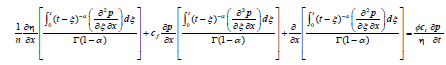The derivative of fractional order is defined as: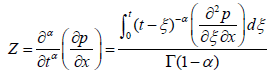With 0 ≤ α < 1. In Eq. (1), Γ (1 − α) is the Euler gamma function, using the definition of Z-value, Eq. (1) may be written as: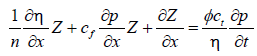Equation (3) is called as the diffusivity equation with memory because it is a combination of the motion equation and the continuity equation. The model is a non-linear and integro-differential form of memory for any axial flow of single phase fluid flow equation for a porous formation.

As a matter of fact, the equation is strictly non-linear because three parts of the equation are non-linear, the first part is non-linear due to dependence of η on pressure, the second part is non-linear since Z is a function of pressure, and the fourth part is a multiplication of porosity, total compressibility, η and pressure derivative with time, therefore, this term also is non-linear.

If there is no thermal equilibrium in the system, a 1D energy balance equations can be written to develop the model equation for describing the temperature propagation in porous media as [14,20], the final form can be written as follow: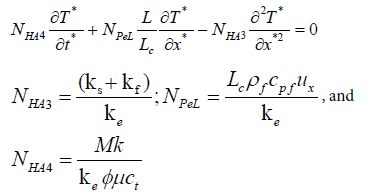Computations on Eq. (4) are carried out for different rock/fluid parameters where the initial and boundary conditions are defined as: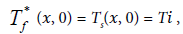in terms of dimensionless form: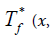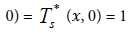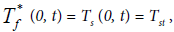in terms of dimensionless form: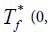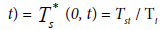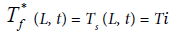, in terms of dimensionless form: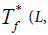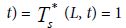Numerical solution

To solve Eq. (4) numerically with the specified initial and boundary conditions, finite difference method with explicit scheme can be used, and then the following equation might be achieved.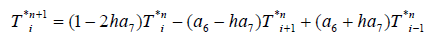Where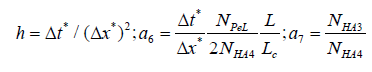In Eq. (5) the local Peclet number can be defined as (NPe)L = Lc ρf cpfum=ke, where Lc represents a characteristic length such as the mean pore throat diameter of the porous medium. During the computation, Lc can be calculated using Winlad’s correlation , Lc = 2πrpt and log rpt = 0.732 + 0.588 log k − 0:864 logϕ, where k is in mD and rpt is in microns. Kolodzie and Pittman [21,22] proposed the preceding correlation as the best permeability estimator for sandstones. Other correlations for carbonate rocks are also proposed in the literature. During the computation of T*, the same procedure is used to calculate the Lc for all the figures .

To solve Eq. (5), the distribution of fluid velocity should be known, which can be obtained using numerical concepts based on the pressure distribution. First, the memory- based diffusivity equation is solved to obtain the reservoir pressures, then the pressure gradient can be determined using finite difference concept, thereafter, the numerical integration is applied to find the distribution of fluid velocity. Finally, the temperature profiles can be calculated based on the fluid velocity distribution [24,25].

#### Results and Discussion

Computations are carried out for a reservoir 1000 m long, where hot water is injected at a constant rate of 17.5 m3 of equivalent water volume per day. All assumed rock and fluid parameters are listed in Table 1. The time and distance steps are set at Δx* = 0.0167 and Δt* = 0.00001. Temperature variation is obtained for the case where the fluid and rock temperatures are equal.

Fluid and rock properties Fluid and rock properties
Cpg =29.7263 [KJ/Kg-K] Sg =20% [vol/vol]
Cpo =2.0934 [KJ/Kg-K] So =60% [vol/vol]
Cps =0.8792 [KJ/Kg-K] Sw =20% [vol/vol]
Cpw =4.1868[KJ/Kg-K] THw =550 K
hc =280.87 [KJ/h-m2-K] Ti =300 K
kg =0.0143 [KJ/h-m-K] ρg =16.7121 [Kg/m3]
ko =1.3962 [KJ/h-m-K] ρo =800.923 [Kg/m3]
ks =9.346 [KJ/h-m-K] ρs =2675.08 [Kg/m3]
kw =3.7758 [KJ/h-m-K] ρw =1000.0 [Kg/ m3]
ki =15 x 10-15 [m2] Φ = 25% [m3/m3]
pi =48263299 [pa] µf =0.12 pa.s [Ns/m2]
cf =1.2473 x 10-9[1/pa] qi =17.5 m3/d [110 bbl/day]
cs =5.8015 x 10-10 [1/pa] A = 300 m × 20 m = 6000 m2

Table 1: Fluid and rock property values for numerical computation.

Variation of reservoir pressure

Equation (3) was solved numerically using space-based derivative concept, initially Darcy diffusivity equation was assumed to be valid and the distribution of the permeability with time and space remain unchanged. In addition, the reservoir formation was considered to be homogeneous and isotropic in its initial phase. All the coefficients are computed at the average pressure of the grid cell ‘i’, at time step ‘n’ .

Figure 1 presents the pressure variation against distance from the wellbore towards the outer boundary of the reservoir. The pressure response increases towards the reservoir boundary after 1 Day, 6 months and 2 years respectively. Minimum formation pressure is found around the wellbore and it gradually increases up to its initial reservoir pressure near to the outer boundary. After 1 day of production, the pressure reaches the initial pressure at almost 20 m from the wellbore whereas it reaches at around 185 and 490 after 6 months and 2 years of the production respectively.

Figure 2 illustrates the variation of pressure with time for the distance of 5 m, 150 m and 500 m from the wellbore towards the boundary of the reservoir using the same Eq. (3). Due to the reservoir depletion, the reservoir pressure decreases as the time goes with more pressure drop away from the wellbore.

Variation of fluid velocity

The fluid velocity with memory effect can be determined based on the pressure distribution. The fluid velocity was calculated using numerical concepts as follow; the pressure distribution was estimated by solving the memory-based diffusivity equation, then finite difference concept was used to determine the pressure gradient, thereafter, the numerical integration was applied to find the distribution of fluid velocity.

Figure 3 illustrates the variations of fluid velocity against distance from the wellbore for 1, 5, 12 and 24 months. Velocities increase and reach its pick near to the wellbore, increasing the production time result in increasing the velocity profile. Velocity has a decreasing trend till reach zero after 100 m, 220 m, 350 m and 540 m after 1, 5, 12 and 24 months respectively. This is due to the fact that velocity has zero value because there is no pressure gradient at these locations. These characteristics clearly identify the extreme memory effect on the reservoir performance around the wellbore and diminish its effect gradually toward the boundary of the reservoir.

Figure 4 depicts the changes of fluid velocity with memory effects over time for 5 m, 20 m, 50 m and 100 m away from the wellbore. In the area near to wellbore, fluid velocity rises very fast and requires significant time to stabilize. The same trend can be observed for 100 m away from wellbore, however there is less velocity compared to 5 m for a particular time.

Variation of reservoir temperature

Figure 5 shows the variation of dimensionless temperature against dimensionless distance. While Figure 6 illustrates the changing of reservoir temperature with respect to distance after 1 month, 5 months,1 year and 2 years. In both figures, the temperature response decreases towards the reservoir boundary after 1 month, 5 months, 1 year and 2 years respectively.

Since hot water is injected at temperature higher than reservoir temperature, so maximum reservoir temperature is found around the wellbore and it gradually decreases to its initial reservoir temperature near to the outer boundary. After 5 month of production, the temperature reaches the initial reservoir temperature at almost 35 m from the wellbore whereas it reaches at around 55 and 70 after 1 year and 2 years of the production respectively.

Figure 7 shows the variation of dimensionless temperature against dimensionless time. While Figure 8 illustrates the changing of reservoir temperature with respect to time at 5 m, 20 m, 50 m and 100 m away from the wellbore. In both figures, the temperature response increases with time due to the heat transfer from hot water to reservoir fluid/ rock, the rate of heat transfer depends on the thermal properties of reservoir rock and fluids; also the fluid velocity has a significant role on the heat diffusion throughout the reservoir.

Usually hot water is injected at temperature higher than reservoir temperature, so sufficient time is required to achieve stability conditions between the injected water and reservoir fluids/rock, i.e. the reservoir temperature approaches to temperature of injected fluid, however, the reservoir temperature will never be equal to the temperature of injected fluid as stated in the thermodynamic. As a result, after 2 years of continuous hot water injection, the reservoir temperature increases to 510 K, 400 K, 310 K, and 305 K at locations of 5 m, 20 m, 50 m, and 100 m respectively.

#### Conclusion

The temperature propagation due to thermal flooding process was investigated based on the implementation of memory concept, a numerical solution of the mathematical models which was developed by Hossain et al. is proposed. Results show that, the distribution of reservoir temperature with respect to time and distance can be estimated by coupling the memory-based equation and the mathematical tool which were developed by Hossain et al., in addition, it can be concluded that, the fluid velocity, time and the rheological properties, have important effects on the temperature distributions throughout the reservoir. In future, the results of the numerical solution can be integrated with lab experiment results to predict performance of thermal flooding process and better understanding of reservoir management.

#### Acknowledgement

The authors are grateful for the support and guidance received from the Deanship of Scientific Research (DSR) at King Fahd University of Petroleum & Minerals (KFUPM).

#### References

Select your language of interest to view the total content in your interested language

### Article Usage

• Total views: 9200
• [From(publication date):
October-2016 - Mar 22, 2019]
• Breakdown by view type
• HTML page views : 9085

## Post your commentCan't read the image? click here to refresh
###### Peer Reviewed Journals

Make the best use of Scientific Research and information from our 700 + peer reviewed, Open Access Journals

International Conferences 2019-20

Meet Inspiring Speakers and Experts at our 3000+ Global Annual Meetings

Top